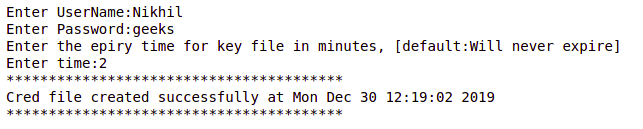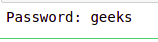Related Articles
Create a Credential file using Python
• Last Updated : 06 Oct, 2020

A credential file is nothing but just a configuration file with a tad bit of encryption and an unseen security structure in the backend. There might be a situation where you might come across these kinds of files while using some kind of cloud platforms. All you do to login to the instance or give the script the permissions to do something without your username and password seems a bit magical but what if you want to do the same? let’s say you have created a script that requires few configurations or credentials for logging in. It is quite irritating for the user to enter the credentials or configurations every time they want to run the code. So what’s the solution? Well, there are many ways to do it but since this is about creating credential files the will use the same method. let your users create a Credentials file or Configurations file that can later be used by the script to get the details it requires. And here’s how to do it.

#### Adding a credential file creator to the script

Really, it’s as simple as copying the below script and adding it in your package but you need to understand a few things if you are looking to get a custom credential or configuration file for your scripts. First, The below script just accepts the user input and add encryption on a few things and write it to a file. If you are looking to add other data, just add a new variable or write it directly to the file. Second, Encryption here is done using Fernet in the cryptography package. So the key is stored in a `.key` file and if you do want to some third party to break the encryption, convert the Credentials creator file to `.exe` or other formats that cannot be read easily.

Note: All the modules used comes built-in with Python, so there is no need to install it externally.

CreateCred.py –

 `#CreateCred.py``#Creates a credential file.``from` `cryptography.fernet ``import` `Fernet``import` `re``import` `ctypes``import` `time``import` `os``import` `sys``  ` `class` `Credentials():``  ` `    ``def` `__init__(``self``):``        ``self``.__username ``=` `""``        ``self``.__key ``=` `""``        ``self``.__password ``=` `""``        ``self``.__key_file ``=` `'key.key'``        ``self``.__time_of_exp ``=` `-``1``  ` `#----------------------------------------``# Getter setter for attributes``#----------------------------------------``   ` `    ``@property``    ``def` `username(``self``):``        ``return` `self``.__username``  ` `    ``@username``.setter``    ``def` `username(``self``,username):``        ``while` `(username ``=``=` `''):``            ``username ``=` `input``(``'Enter a proper User name, blank is not accepted:'``)``        ``self``.__username ``=` `username``  ` `    ``@property``    ``def` `password(``self``):``        ``return` `self``.__password``  ` `    ``@password``.setter``    ``def` `password(``self``,password):``        ``self``.__key ``=` `Fernet.generate_key()``        ``f ``=` `Fernet(``self``.__key)``        ``self``.__password ``=` `f.encrypt(password.encode()).decode()``        ``del` `f``  ` `    ``@property``    ``def` `expiry_time(``self``):``        ``return` `self``.__time_of_exp``  ` `    ``@expiry_time``.setter``    ``def` `expiry_time(``self``,exp_time):``        ``if``(exp_time >``=` `2``):``            ``self``.__time_of_exp ``=` `exp_time``  ` `  ` `    ``def` `create_cred(``self``):``        ``"""``        ``This function is responsible for encrypting the password and create  key file for``        ``storing the key and create a credential file with user name and password``        ``"""``  ` `        ``cred_filename ``=` `'CredFile.ini'``  ` `        ``with ``open``(cred_filename,``'w'``) as file_in:``            ``file_in.write(``"#Credential file:\nUsername={}\nPassword={}\nExpiry={}\n"``            ``.``format``(``self``.__username,``self``.__password,``self``.__time_of_exp))``            ``file_in.write(``"++"``*``20``)``  ` `  ` `        ``#If there exists an older key file, This will remove it.``        ``if``(os.path.exists(``self``.__key_file)):``            ``os.remove(``self``.__key_file)``  ` `        ``#Open the Key.key file and place the key in it.``        ``#The key file is hidden.``        ``try``:``  ` `            ``os_type ``=` `sys.platform``            ``if` `(os_type ``=``=` `'linux'``):``                ``self``.__key_file ``=` `'.'` `+` `self``.__key_file``  ` `            ``with ``open``(``self``.__key_file,``'w'``) as key_in:``                ``key_in.write(``self``.__key.decode())``                ``#Hidding the key file.``                ``#The below code snippet finds out which current os the scrip is running on and does the taks base on it.``                ``if``(os_type ``=``=` `'win32'``):``                    ``ctypes.windll.kernel32.SetFileAttributesW(``self``.__key_file, ``2``)``                ``else``:``                    ``pass``  ` `        ``except` `PermissionError:``            ``os.remove(``self``.__key_file)``            ``print``(``"A Permission error occurred.\n Please re run the script"``)``            ``sys.exit()``  ` `        ``self``.__username ``=` `""``        ``self``.__password ``=` `""``        ``self``.__key ``=` `""``        ``self``.__key_file``  ` `  ` `def` `main():``  ` `    ``# Creating an object for Credentials class``    ``creds ``=` `Credentials()``  ` `    ``#Accepting credentials``    ``creds.username ``=` `input``(``"Enter UserName:"``)``    ``creds.password ``=` `input``(``"Enter Password:"``)``    ``print``(``"Enter the epiry time for key file in minutes, [default:Will never expire]"``)``    ``creds.expiry_time ``=` `int``(``input``(``"Enter time:"``) ``or` `'-1'``)``  ` `    ``#calling the Credit``    ``creds.create_cred()``    ``print``(``"**"``*``20``)``    ``print``(``"Cred file created successfully at {}"``    ``.``format``(time.ctime()))``  ` `    ``if` `not``(creds.expiry_time ``=``=` `-``1``):``        ``os.startfile(``'expire.py'``)``  ` `  ` `    ``print``(``"**"``*``20``)``  ` `if` `__name__ ``=``=` `"__main__"``:``    ``main()`

Output:#### Reading the credential file

To read the credential, it is as simple as reading a normal file using the python file reading methodologies but to decrypt the data you need to have the key that is used for encryption. So the Credentials file creator creates both a credential file and a key file. The retrieval script uses the key file and decrypts the data.

 `#Retrive credentials.`` ` `from` `cryptography.fernet ``import` `Fernet``import` `os`` ` `cred_filename ``=` `'CredFile.ini'``key_file ``=` `'key.key'`` ` `key ``=` `''`` ` `with ``open``(``'key.key'``,``'r'``) as key_in:``    ``key ``=` `key_in.read().encode()`` ` `#If you want the Cred file to be of one ``# time use uncomment the below line``#os.remove(key_file)`` ` `f ``=` `Fernet(key)``with ``open``(cred_filename,``'r'``) as cred_in:``    ``lines ``=` `cred_in.readlines()``    ``config ``=` `{}``    ``for` `line ``in` `lines:``        ``tuples ``=` `line.rstrip(``'\n'``).split(``'='``,``1``)``        ``if` `tuples[``0``] ``in` `(``'Username'``,``'Password'``):``            ``config[tuples[``0``]] ``=` `tuples[``1``]`` ` `    ``passwd ``=` `f.decrypt(config[``'Password'``].encode()).decode()``    ``print``(``"Password:"``, passwd)`

Output:And now if you have read the scripts properly you might have seen a file ‘expire.py’. This script starts a clock when the credential file is created and deletes the key file so that decryption is no longer possible when the specified time is over.

expire.py
 `import` `os``import` `time``  ` ` ` `key_file ``=` `'key.key'``key_exp_start ``=` `time.time()``cred_filename ``=` `'CredFile.ini'``  ` `with ``open``(cred_filename, ``'r'``) as cred_in:``  ` `    ``lines ``=` `cred_in.readlines()``    ``config ``=` `{}``    ``for` `line ``in` `lines:``        ``tuples ``=` `line.rstrip(``'\n'``).split(``'='``, ``1``)``         ` `        ``if` `tuples[``0``] ``in` `(``'Expiry '``):``            ``config[tuples[``0``]] ``=` `tuples[``1``]``              ` `    ``if` `not``(config[``'Expiry '``] ``=``=` `-``1``):``  ` `        ``# Time below is in seconds.``        ``time_for_exp ``=` `int``(config[``'Expiry '``]) ``*` `60``         ` `        ``while``(os.path.isfile(key_file)):``            ``time.sleep(``10``)``             ` `            ``if` `(``not``(time.time() ``-` `key_exp_start <``=` `time_for_exp) ``                ``and` `os.path.isfile(key_file)):``                ``os.remove(key_file)`

Attention geek! Strengthen your foundations with the Python Programming Foundation Course and learn the basics.

To begin with, your interview preparations Enhance your Data Structures concepts with the Python DS Course.

My Personal Notes arrow_drop_up